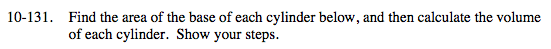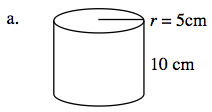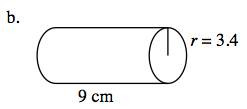### Home > MC2 > Chapter 10 > Lesson 10.2.5 > Problem10-131

10-131.The base is a circle, so find the area of the circle using the following equation:
Area= π(r ²)

Area = π(52)

Area = 25π cm² or approximately 78.54 cm².
Now use the equation for the volume of a cylinder:
Volume = (area of base) (height)

Volume = (78.54)(10)

785.4 cm3See part (a).